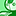QlikView App Dev

Discussion Board for collaboration related to QlikView App Development.

Announcements
QlikWorld 2022, LIVE in Denver CO., May 16-19, 2022. REGISTER NOW TO RECEIVE EARLY BIRD PRICING
cancel
Showing results for
Did you mean:Creator

Moving Calculated Dimension to Set Analysis

All,

I have a calculated dimension as such :

=if(MATURITY_DATE >= MonthStart(SUMMARY_DATE) and MATURITY_DATE <= SUMMARY_DATE,'Matured','')

and a expression as:

sum({\$<SUMMARY_DATE = ,UK = {'N'}, FRUIT = {' '}>} PRICE)/-1000000

This gives me what I want which is what portion of my produce has matured.  I need to move the calculated dimension in to my expression due to the way I need to report (I have created a list of values with ValueList('Total','Maturites'))

When I move my calculated field on to my expression as:

sum({\$<SUMMARY_DATE = ,UK= {'N'}, FRUIT = {' '},MATURITY_DATE = {">=\$(SUMMARY_DATE)<\$(SUMMARY_DATE)"}>} PRICE)/-1000000

It doesn't seem to give me what I want.  Maturity date is in number format, Summary date is in date.  I wonder if that is the reason.

Any help is more than appreciated.

Thanks,

Labels (1)
• expression

1 Solution

Accepted SolutionsPartner
Thats true indeed. I make sure to always use nums to be sure it is going to work. Also make sure there is no time with that date. So always use floor.
3 RepliesPartner
if one is a num and one is a date this would cause an issue yes. so its best to change this and see if it works.Creator
Author
It is strange that is not an issue within the calculated dimension, but in the set analysis.Partner
Thats true indeed. I make sure to always use nums to be sure it is going to work. Also make sure there is no time with that date. So always use floor.Community Browser